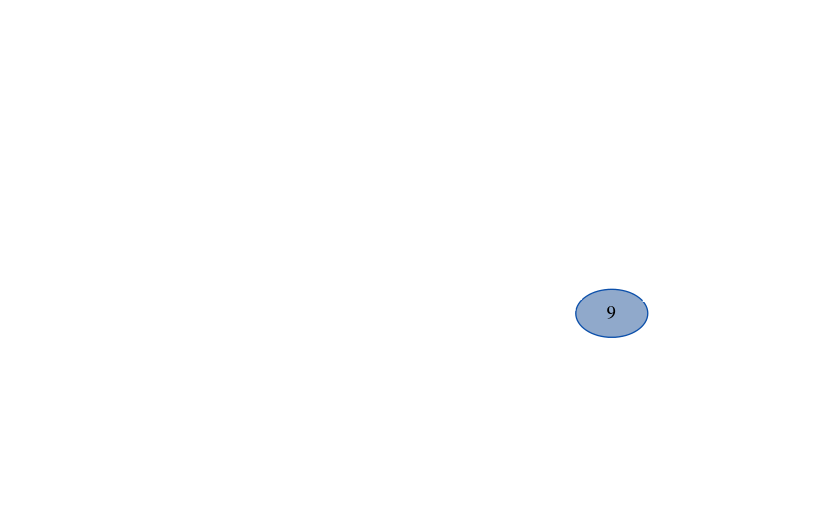## Inductive Graphs (Functional Graph Library)

Tikhon Jelvis (tikhon@jelv.is)

Zoom in/out: Ctrl or Command + +/-

# 1 Graphs

• graphs are tricky in functional programming
• but what's easy?
• lists
• trees

# 2 Pattern Matching

• why?
• we can pattern match on trees and lists!
```foo []     = []
foo (x:xs) = bar x : foo xs
```
• computation follows the "shape" of the type

# 3 Inductive Data Types

• lists have exactly one decomposition at any given point
• reverse of how they are constructed
• "inductive data types":
```data [a] = []      -- base case
| a : [a] -- recursive case
```

# 4 Inductive Graphs?

• can we do the same for graphs?
• no
• a graph does not have a canonical decomposition
• multiple ways to construct the same graph

# 5 Inductive Graphs!

• we can give up on canonicity
• view graphs inductively
• multiple possible views
• graphs aren't actually built this way
• library: fgl

# 6 Type

• decompose graph into:
• a node
• its edges
• the rest of the graph
```data Graph =
Empty
| (Context [Edge] Node [Edge]) :& Graph
```
• ignoring node/edge labels

# 7 Demo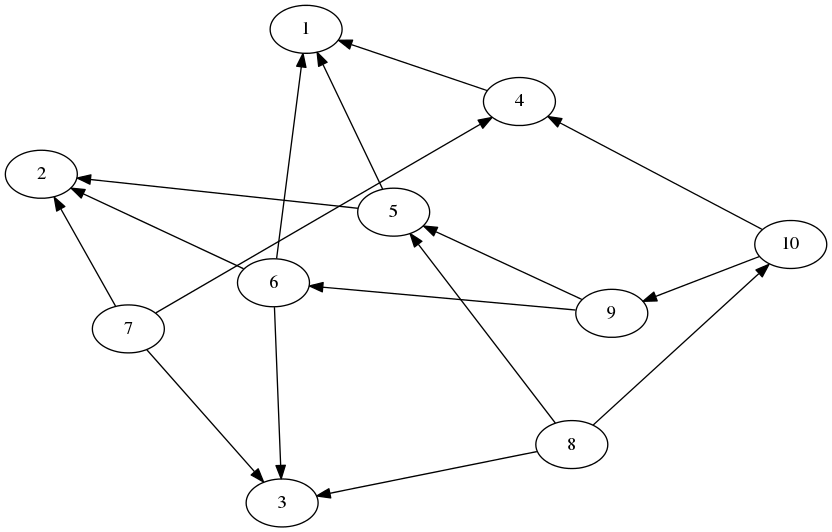# 8 Demo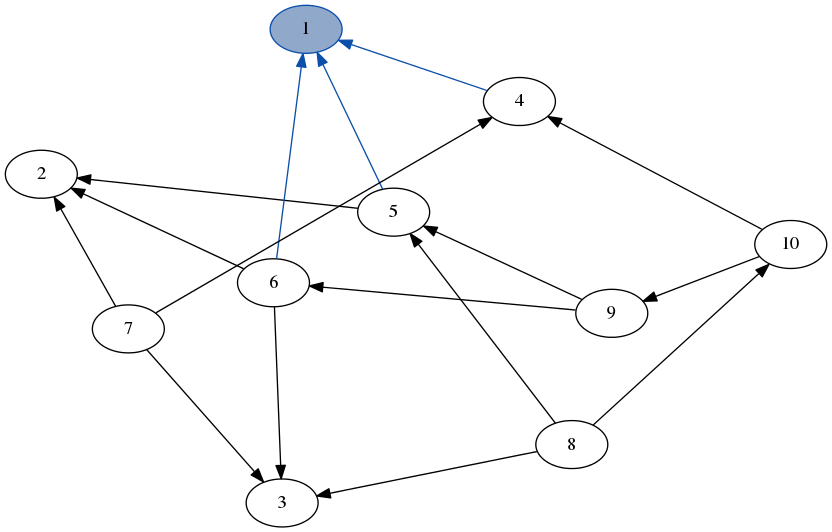# 9 Demo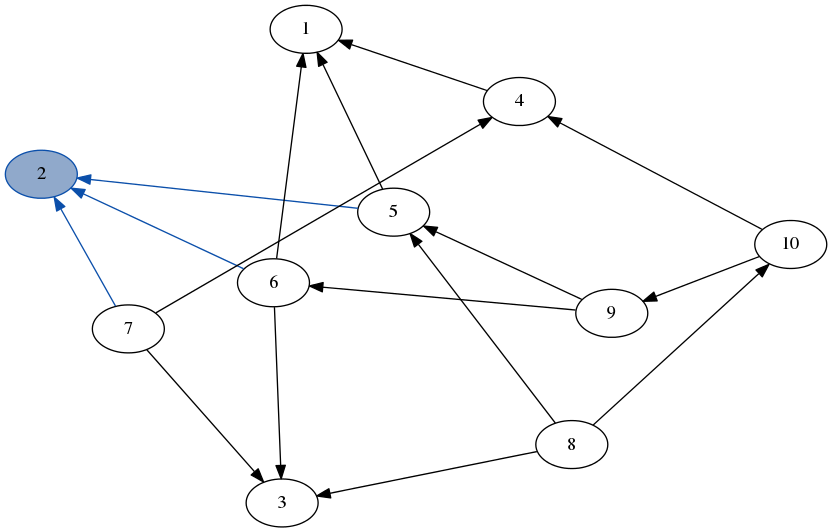# 10 Demo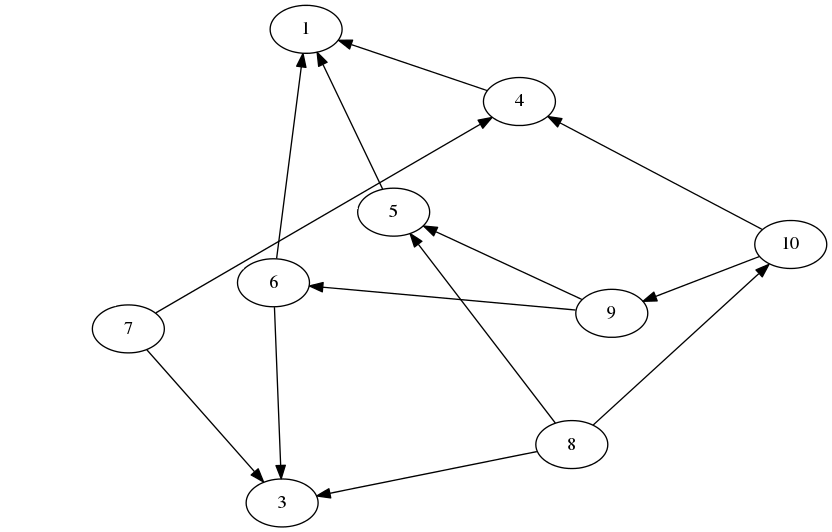# 11 DFS

• a depth-first search, producing a list of nodes:
```dfs (x:xs) (match x -> (Just ctx, g)) =
x : dfs (neighbors' ctx ++ xs) g

dfs (_:xs) graph = dfs xs graph
```
• base cases: empty stack or empty graph

# 12 DFS

-stack: ``# 13 DFS

-stack: `[5, 4, 6]`# 14 DFS

-stack: `[2, 8, 9, 4, 6]`# 15 DFS

-stack: `[7, 6, 8, 9, 4, 6]`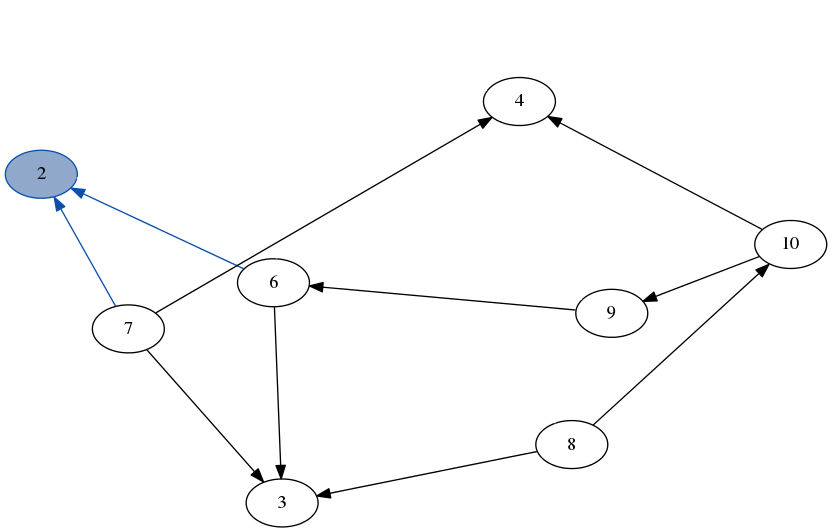# 16 DFS

-stack: `[4, 3, 6, 8, 9, 4, 6]`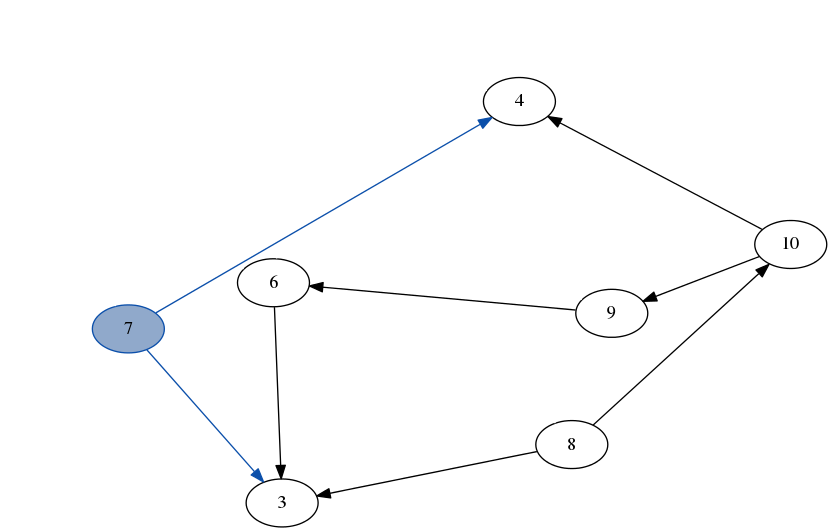# 17 DFS

-stack: `[10, 4, 3, 6, 8, 9, 4, 6]`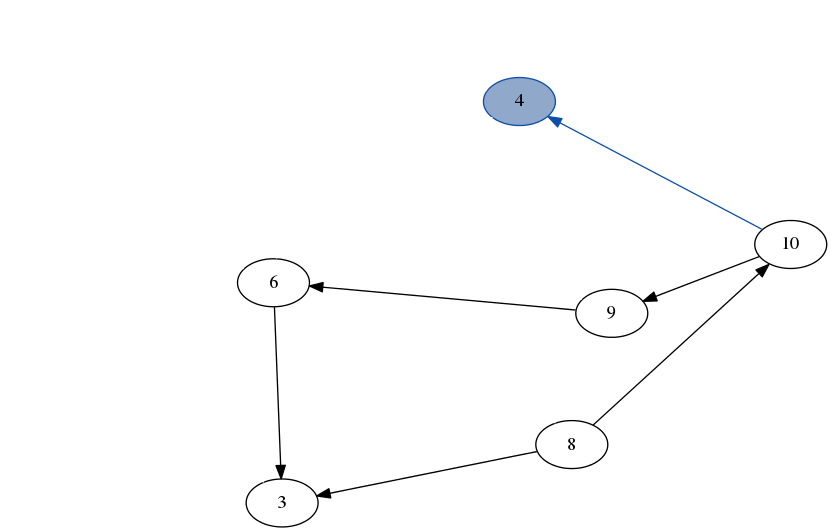# 18 DFS

-stack: `[8, 9, 4, 3, 6, 8, 9, 4, 6]`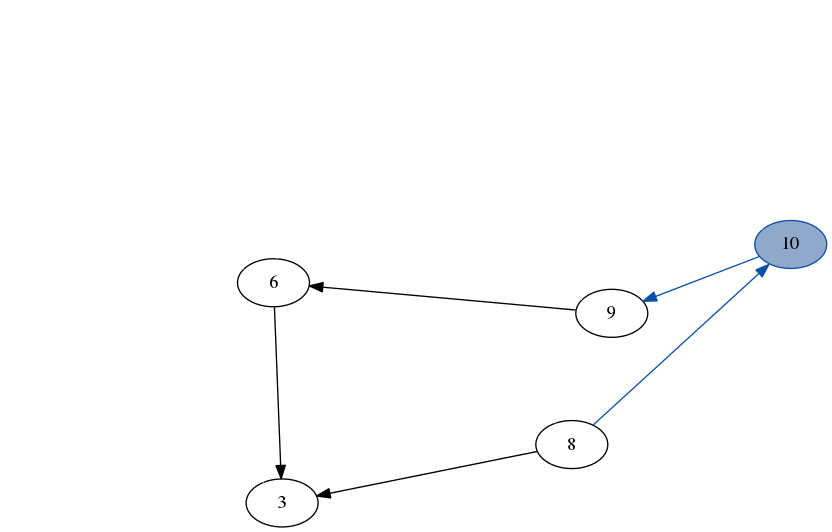# 19 DFS

-stack: `[3, 9, 4, 3, 6, 8, 9, 4, 6]`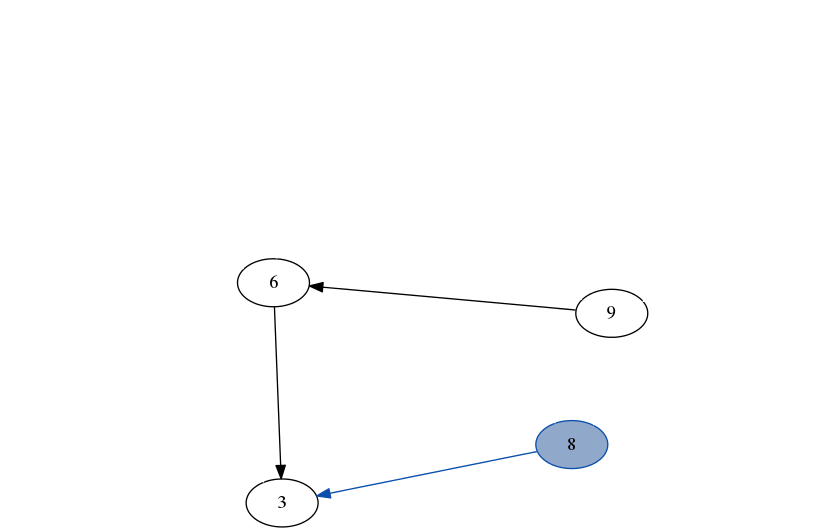# 20 DFS

-stack: `[6, 9, 4, 3, 6, 8, 9, 4, 6]`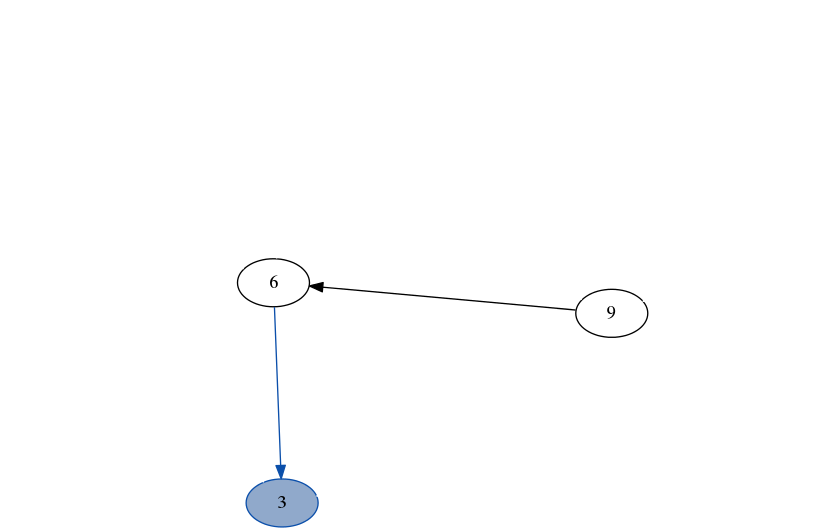# 21 DFS

-stack: `[9, 9, 4, 3, 6, 8, 9, 4, 6]`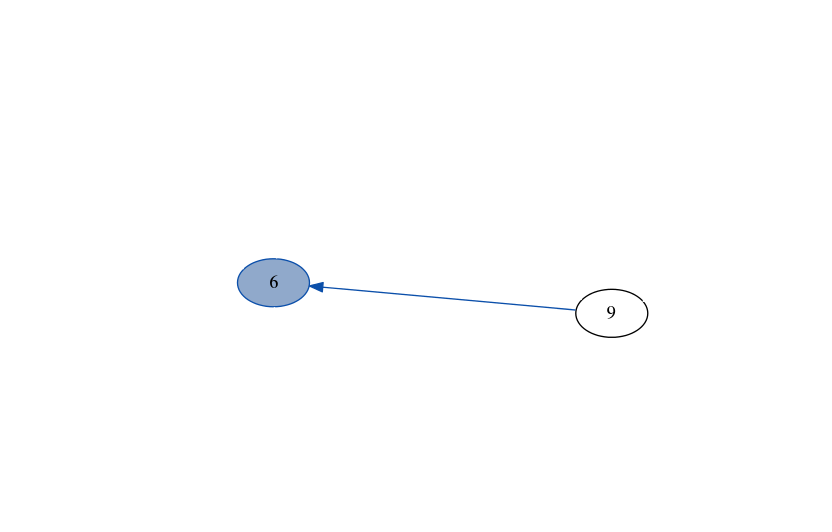# 22 DFS

-stack: `[9, 4, 3, 6, 8, 9, 4, 6]`